Select Page

Is it enough? Dayenu.It would have been enough that the Creator gave us 27 Essential Forces or pressure mediations to create our universe. It would have been enough that He gave us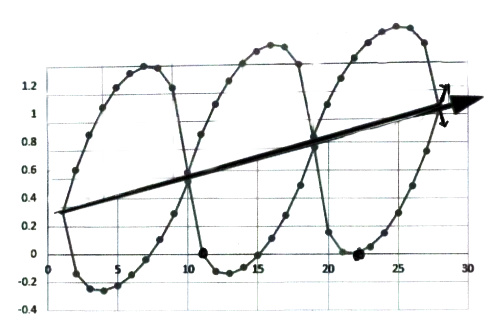the 27 Hebrew letters to represent them so that we may interact with them and one day comprehend them. It would have been enough that He gave numerical values to those 27 letters according to a sine wave with a slope of 1.6 and an exponential scale (y = 1.313x) that is based on the year He also gave us the Torah, 1313 BCE. For anyone that has not been following this series from its inception, you can find the entire work here.

Dayenu.

It would have been enough that He arranged the Alef-Bet so that the 27 letters times the slope of 1.6 equaled 43.2, the frequency (432 Hz) of the shape of the Tree-of-Life.Two stacked cubes with the length of Vav(ו) per side for a volume of 63 each cube, total 432.

Dayenu.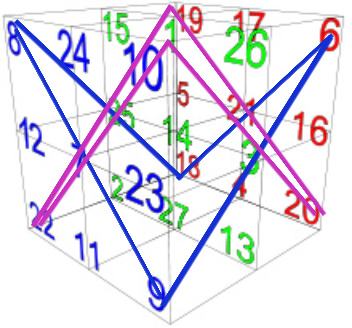It would have been enough that He gave us the 42-Letter Names comprised of those letters to let us access those forces. It would have been enough that He arranged those 27 letters into a tight 33 cube so that each and every one of the resultant 27 columns and rows in that cube sums to 42 and connects us to those Names. It would have been enough that He arranged the 28 diagonals of that cube so that they summed in pairs and collectively to multiples of 42, or that the ordinal value of the entire cube is 9 x 42. It would have been enough that He arranged that the cube’s corners, alternatively the end points of those diagonals to form opposing triangles that when multiplied form multiples of 42 (i.e.  8 x 9 and 9 x 6 = 126; and 22 x 10 and 10 x 20 = 420) and the Masonic symbol of the compass.

Dayenu.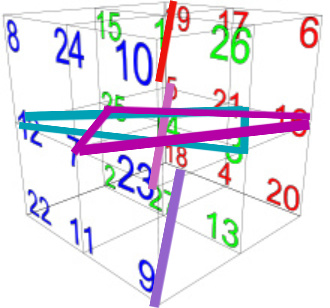It would have been enough that He arranged each letter in the cube so that they formed triangles and bars of numerical triplets from 111999. It would have been enough that He arranged those numerical triplets into parallel Stars of David along a central axis of the cube. It would have been enough that He arranged all 27 letters so that they formed 9 triplet triangles and bars in order of the Alef-bet (…,דהו) ( ,אבג). It would have been enough that He arranged those 9 triplets so that they formed parallel Stars of David and bars along yet another central axis of the cube, perpendicular to the first one. It have would have been enough that He arranged all 3 orthogonal axes so that they formed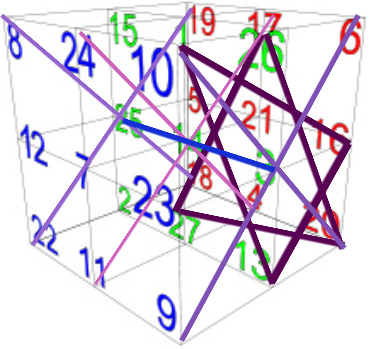9 parallel Stars of David and crosshairs, and made each axis set of Stars and crosshairs equal to 6660, resulting in all of them together equaling 3 x 6660 and 4 x 4995, the value of the entire Alef-bet.  It would have been enough that He arranged for all the 36 letters utilized in each of those parallel sets of Stars of David and crosshairs that equal 6660 to be equal to the sum of the integers from 1 – 36,  or (666 x 10).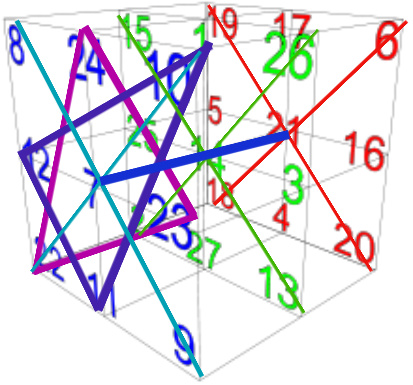Dayenu.

It would have been enough that He arranged each letter in the cube so that they formed triangles and bars of numerical triplets that generated the triplet root letters of every Hebrew word, and that the entire Hebrew language thus formed a tangled Bird’s Nest within the confines of the cube.

Dayenu.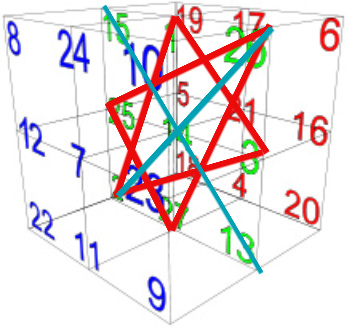It would have been enough that He arranged for specific triangles and/or Stars of David to give us specific key dates in history, including the date of the Flood twice in 1656 HC and 1657 HC; the infamous 9/11/2001 that may have signaled the start of Oy and Ashrei;  the construction of the Second Holy Temple in 3408 HC; and the year 1776, whether CE or HC.

Dayenu.

It would have been enough that He arranged the cube so that each tier of 9 sub-cubes or letters summed to 1665, and that the year of the Flood in 1656 HC equals (16659).

Dayenu.

It would have been enough that He arranged the cube so that the left face, or alternatively the back tier of the 3-14-25 axis, equals 1395, the exact sum of the numerical values of the 6 Matriarchs—Sarah, Leah, Rivka, Eve, Miriam, and Rachel—and that the Star and crosshairs of the right face, or alternatively the front face of that same axis, equals 1620, which is the sum of their complete numerical values, representing Binah (1000) and Keter (620), and also the resultant value of (4 x 9 x 9 x 5).

Dayenu.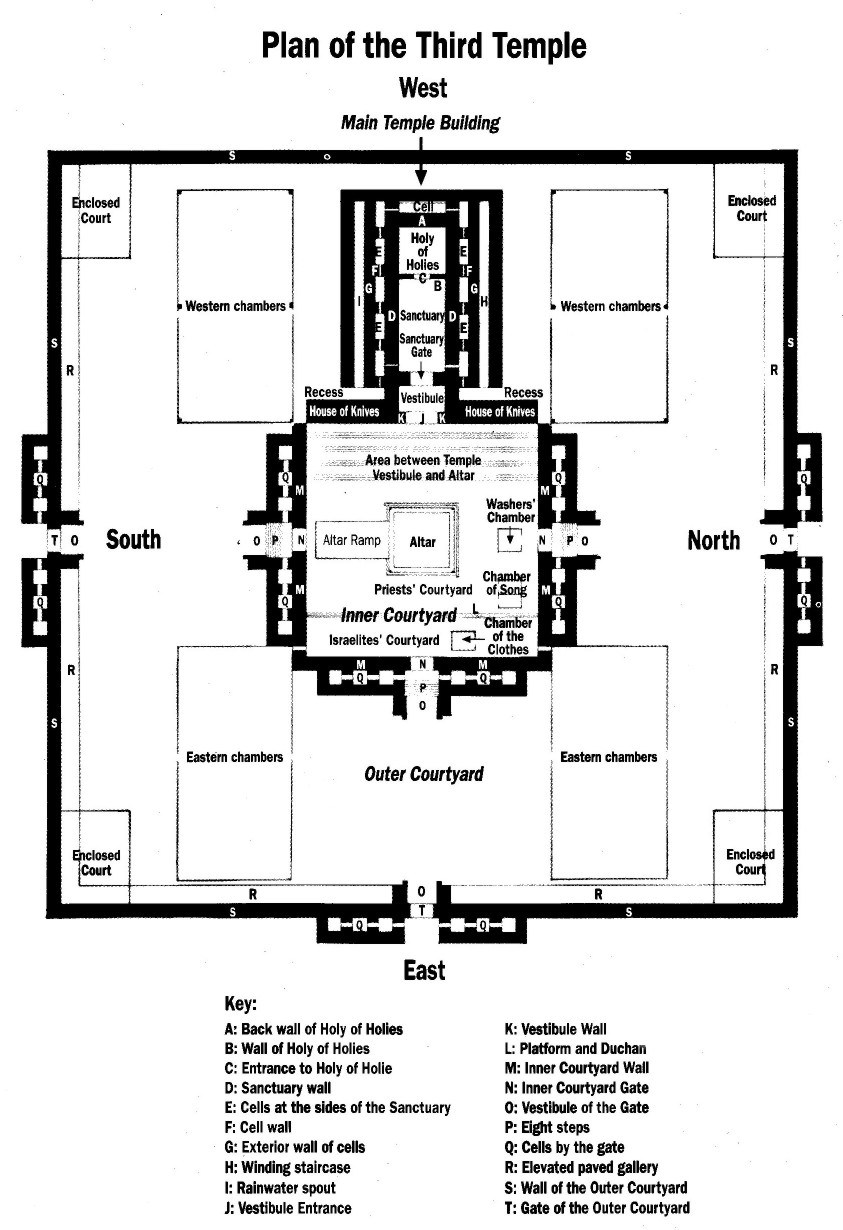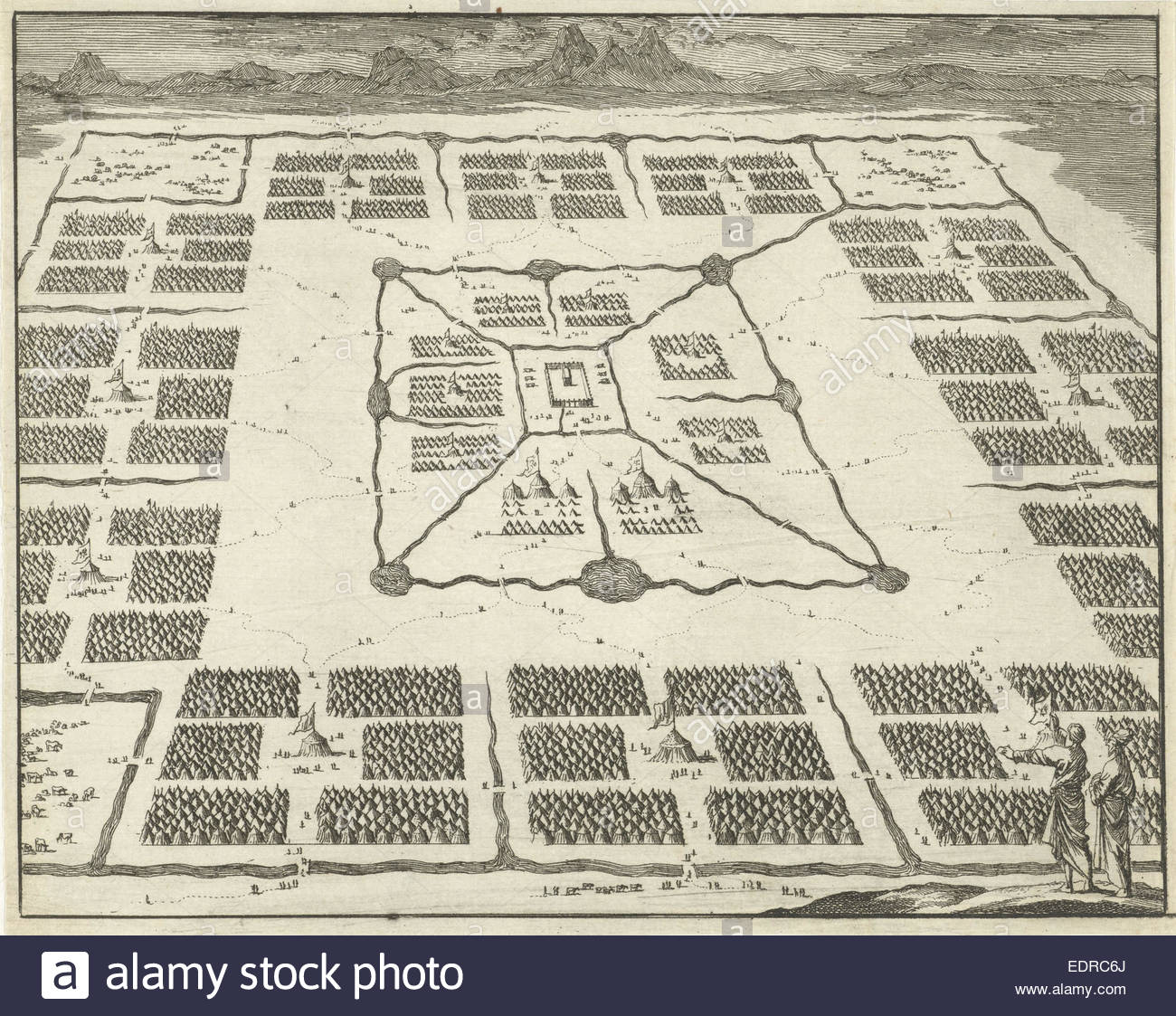It would have been enough that He arranged the cube to compliment and fit into the exact center of the 112 Holy Triplets, which He had concealed throughout the Torah. It would have been enough that He arranged the cube so that the 8 corners summed to 112, and that each pair of opposite corners equaled 28.  It would have been enough that He arranged the cube so that the sum of the ordinal values of central tier of the cube without the central number/cube of 14 is 112, and so that the sum of all 3 tiers without the central Pi (3 – 14 – 25) column is 336, the amount of letters in the 112 Triplets.  It would have been enough that He arranged the cube so that it formed 6 internal pyramids centered on the central 14 cube that fit seamlessly together with a total ordinal value of 420, and whose 24 pyramid corners add up to 336. It would have been enough that He arranged the cube so that the letter total without that central Pi (3 – 14 – 25) column is 4242.

Dayenu.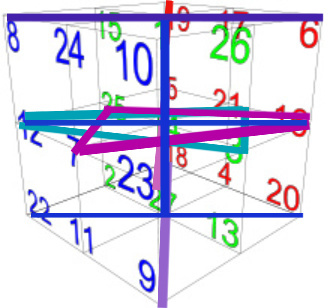It would have been enough that He arranged the cube so that each horizontal tier equaled 1665, exactly 1/3 of the Alef-bet (4995). It would have been enough that He arranged the cube so that any two of those 3 tiers equaled 3330, as in the 3330, or 66.6 jubilee, years from the reception of the Torah at Mt Sinai in 2448 HC and today in 5778 HC. It would have been enough that He arranged the cube so that the 3 parallel Stars of David in the vertical axis totaled 1665 and the crosshairs or diagonals totaled 3330, splitting the cube exactly into 1/3 and 2/3.

Dayenu.

It would have been enough that He arranged the cube so that there were 6 faces of 9 cubes each and that He placed the numerical string  …999999… at the 761st digit in Pi and the sequence …4995… representing the 27 letters of the Alef-bet at the 777th digit and ending with the 780th digit, considering the full sequence is …999999837297804995  and that 729 equals 93 and considering that the 666th digit in Pi is …343… or 73. It would have been enough that He arranged the Alef-bet and cube so that 4995 = (45 x 111) with 111 being the value of Alef (א), One, and 45 being representative of Adam, of an aspect of the YHVH associated with Man, and of the 45th and current President of the USA.

Dayenu.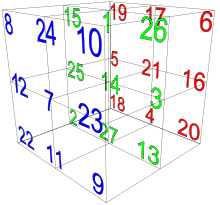It would have been enough that He arranged the cube so that His Name Yah (יה), or 15, follows a progression from 1 to 10 to 100 in: a parallel set of diagonals in the vertical (1 – 14 – 27) axis, which  breaks down tier by tier to 151501500; and along the (7 – 14 – 21) axis as a triangle, cross bar, and triangle that also equal 151501500 respectively; and likewise along the (3 – 14 – 25) axis, where a triangle, cross bar, and triangle also equal 151501500 respectively. It would have been enough that He arranged the cube so that His Name Yah (יה) x Alef (א) or (15 x 111) = 1665 was built into it in so many ways. It would have been enough that He arranged the cube so that the vertical (1 – 14 – 27) axis also contained the 151501500 progression in multiple other ways with the uppermost tier having a triangle of 150, another of 1500, and a bar of 15; the middle tier having a triangle of 15, and another one of 1500, for a total of 1515; and the bottommost tier having a triangle of 15, another of 150, and a bar of 1500.

Dayenu.

It would be enough if He had arranged the cube so that the left face, or alternatively the back tier of the (3-14-25) axis, equals 1395, which like the sum of the 6 Matriarch is the sum numerical value of the 6 Patriarchs—Abraham, Isaac, Jacob, Joseph, Aaron, and Moses.  It would be enough if He had arranged the cube so that so many of its resultant values equaled 1665, the complete value of those same 6 Patriarchs.   It would be enough if He had arranged the cube so that it reflected the numerical value of the names of the 12 Matriarchs and Patriarchs as collectively 3285, and with the addition of Adam, 45, the 13 would equal 3330.

Dayenu.

It would have been enough that He arranged the cube so that the rear face, or vertical tier, equaled 855, the location in Pi (855th digit) where we find the digitalstring …1000… that split the first 1000 digits into the sum of the 11 sefirot (3804) and 666. It would have been enough that He arranged the 4 Essential Elements of Creation that comprise the 112 Triplets so that that each connects to 1000, or the 103 cube of Binah: the 1000 letters of the Shema ; the sum of the square roots of the 72 Names that sum to 1000.0; the difference between the Torah’s first verse Bereshit 1:1 and the 42-Letter Name, (3701 -2701) = 1000.

Dayenu.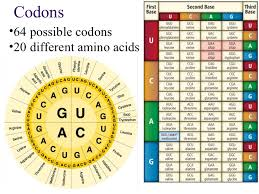It would have been enough that He arranged the cube so that the central cube that made it all work was associated with David (14) and with the letter Nun (נ) of numerical value 50, linking us to the 50 Gates of Binah associated with the jubilee year, and the necessary connection between Da’at, the 11th sefira, and Moshiach Ben David (424 + 50) = 474. It would have been enough that He arranged the cube so that the central cube that made it all work had an ordinal value of 14 and the letter value 50, as in the (50 + 14) = 64 codons (Triplets) in our DNA, and as in Adam and Eve (45 + 19) = 64, the two sources of life provided by the Creator. It would have been enough had 64 not also been a cube of 43.

Dayenu.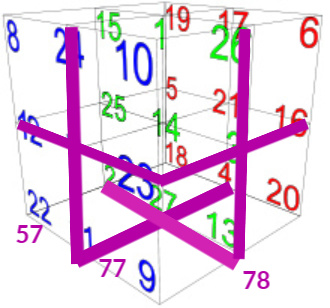It would have been enough that He arranged the cube so that the sum of the crosshatches of 3 contiguous faces gives us 5778: the bottom face (2,4,13,11,27) is 57, the front face (12,24,23,11,7) is 77 and the right face (23,26,16,13,3) is 78. It would have been enough that He arranged the cube so that the complete set of crosshatches (417), with the corners (112) and the center cube (14) or (417 + 126) = 543, the numerical value of “I am that I am (אהיה־אשר־אהיה),” considering there is the Pi (3 – 14 – 25) triplet bar through the heart of the cube that could represent the small gematria (3142) of  “I am that I am,” and considering the Triplet asher (אשר) has an ordinal value of 42 like the two Ehyeh (אהיה).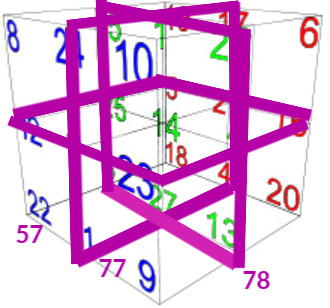Dayenu.

It would have been enough that He arranged the cube so that each tier and several sets of parallel bars had not totaled 126 or (3 x 42).  It would have been enough that He arranged the cube so that each of the 6 faces surrounding the central cube of 14, the numerical value of David (דוד), each totaled 126, the complete numerical value of King, Melech (מלך).  It would have been enough that He arranged time so that the midpoint in King David’s life (2889 HC) was also the midpoint from Adam to 5778, given that He placed David’s cube in the center of the 27 cubes and 14 is the middle number from 1 – 27.  It would have been enough that He gave us King David, who was born in 2954 HC, given that 2954 = (141 + 142 + 143). It would have been enough that David was 35 years old at the midpoint in his life in 2889 and that the 35th of the 72 Names (כוק) has a value of 126, and that Israel just formed its 35th Government after 500 days, 3 elections and both 70 (last government) and 72 years, given that there is a triplet bar sequence in the cube (23 – 3 – 16) or in letter values (500370).

Dayenu. Dayenu (דינו), represents the 6 (ו)-sided cube of ordinal value 14 (די) and Hebrew letter (נ).

Dayenu. Dayenu

For anyone that has not been following this series from its inception, you can find the entire work here.